# 14.E: Multiple Integration (Exercises)

$$\newcommand{\vecs}{\overset { \rightharpoonup} {\mathbf{#1}} }$$ $$\newcommand{\vecd}{\overset{-\!-\!\rightharpoonup}{\vphantom{a}\smash {#1}}}$$$$\newcommand{\id}{\mathrm{id}}$$ $$\newcommand{\Span}{\mathrm{span}}$$ $$\newcommand{\kernel}{\mathrm{null}\,}$$ $$\newcommand{\range}{\mathrm{range}\,}$$ $$\newcommand{\RealPart}{\mathrm{Re}}$$ $$\newcommand{\ImaginaryPart}{\mathrm{Im}}$$ $$\newcommand{\Argument}{\mathrm{Arg}}$$ $$\newcommand{\norm}{\| #1 \|}$$ $$\newcommand{\inner}{\langle #1, #2 \rangle}$$ $$\newcommand{\Span}{\mathrm{span}}$$ $$\newcommand{\id}{\mathrm{id}}$$ $$\newcommand{\Span}{\mathrm{span}}$$ $$\newcommand{\kernel}{\mathrm{null}\,}$$ $$\newcommand{\range}{\mathrm{range}\,}$$ $$\newcommand{\RealPart}{\mathrm{Re}}$$ $$\newcommand{\ImaginaryPart}{\mathrm{Im}}$$ $$\newcommand{\Argument}{\mathrm{Arg}}$$ $$\newcommand{\norm}{\| #1 \|}$$ $$\newcommand{\inner}{\langle #1, #2 \rangle}$$ $$\newcommand{\Span}{\mathrm{span}}$$$$\newcommand{\AA}{\unicode[.8,0]{x212B}}$$

## 13.1: Iterated Integrals and Area

### Terms and Concepts

1. When integrating $$f_x(x,y)$$ with respect to x, the constant of integration C is really which: $$C(x)\text{ or }C(y)$$? What does this mean?

2. Integrating an integral is called _________ __________.

3. When evaluating an iterated integral, we integrate from _______ to ________, then from _________ to __________.

4. One understanding of an iterated integral is that $$\displaystyle \int_a^b \int_{g_1(x)}^{g_2(x)}\,dy\,dx$$ gives the _______ of a plane region.

### Problems

In Exercises 5-10, evaluate the integral and subsequent iterated integral.

5.
(a) $$\displaystyle \int_2^5 (6x^2+4xy-3y^2)\,dy$$
(b) $$\displaystyle \int_{-3}^2 \int_2^5 (6x^2+4xy-3y^2)\,dy\,dx$$

6.
(a) $$\displaystyle \int_0^\pi (2x\cos y +\sin x)\,dx$$
(b) $$\displaystyle \int_{0}^{\pi/2} \int_0^\pi (2x\cos y +\sin x)\,dx\,dy$$

7.
(a) $$\displaystyle \int_1^x (x^2y-y+2)\,dy$$
(b) $$\displaystyle \int_0^2 \int_1^x (x^2y-y+2)\,dy\,dx$$

8.
(a) $$\displaystyle \int_y^{y^2} (x-y)\,dx$$
(b) $$\displaystyle \int_{-1}^1 \int_y^{y^2} (x-y)\,dx\,dy$$

9.
(a) $$\displaystyle \int_0^{y} (\cos x \sin y)\,dx$$
(b) $$\displaystyle \int_0^\pi \int_0^{y} (\cos x \sin y)\,dx\,dy$$

10.
(a) $$\displaystyle \int_0^{x} \left (\frac{1}{1+x^2}\right )\,dy$$
(b) $$\displaystyle \int_1^2 \int_0^{x} \left (\frac{1}{1+x^2}\right )\,dy\,dx$$

In Exercises 11-16, a graph of a planar region $$R$$ is given. Give the iterated integrals, with both orders of integration $$dy\,dx$$ and $$dx\,dy$$, that give the area of $$R$$. Evaluate one of the iterated integrals to find the area.

11.12.13.14.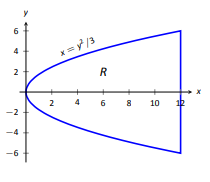15.16.In Exercises 17-22, iterated integrals are given that compute the area of a region R in the $$xy$$-plane. Sketch the region R, and give the iterated integral(s) that give the area of R with the opposite order of integration.

17. $$\displaystyle \int_{-2}^2 \int_0^{4-x^2}\,dy\,dx$$

18. $$\displaystyle \int_{0}^1 \int_{5-5x}^{5-5x^2}\,dy\,dx$$

19. $$\displaystyle \int_{-2}^2 \int_0^{2\sqrt{4-y^2}}\,dx\,dy$$

20. $$\displaystyle \int_{-3}^3 \int_{-\sqrt{9-x^2}}^{\sqrt{9-x^2}}\,dy\,dx$$

21. $$\displaystyle \int_{0}^1 \int_{-\sqrt{y}}^{\sqrt{y}}\,dx\,dy +\int_1^4 \int_{y-2}^{\sqrt{y}}\,dx\,dy$$

22. $$\displaystyle \int_{-1}^1 \int_{(x-1)/2}^{(1-x)/2}\,dy\,dx$$

## 13.2: Double Integration and Volume

### Terms and Concepts

1. An integral can be interpreted as giving the signed area over an interval; a double integral can be interpreted as giving the signed ________ over a region.

2. Explain why the following statement is false: "Fubini's Theorem states that $$\int_a^b \int_{g_1(x)}^{g_2(x)}f(x,y)\,dy\,dx = \int_a^b \int_{g_1(y)}^{g_2(y)}f(x,y)\,dx\,dy$$."

3. Explain why if $$f(x,y)>0$$ over a region R, then $$\int\int_R f(x,y)\,dA >0$$.

4. If $$\int\int_R f(x,y)dA= \int\int_R g(x,y)\,dA$$, does this imply $$f(x,y)=g(x,y)$$?

### Problems

In Exercises 5-10,
(a) Evaluate the given iterated integral, and
(b) rewrite the integral using the other order of integration.

5. $$\int_1^2 \int_{-1}^1 \left ( \frac{x}{y}+3\right )\,dx\,dy$$

6. $$\int_{-\pi/2}^{\pi/2} \int_{0}^\pi (\sin x \cos y$$\,dy\,dx\)

7. $$\int_0^4 \int_{0}^{-x/2+2} \left ( 3x^2-y+2\right )\,dy\,dx$$

8. $$\int_1^3 \int_{y}^3 \left ( x^2y-xy^2\right )\,dx\,dy$$

9. $$\int_0^21\int_{-\sqrt{1-y}}^{\sqrt{1-y}}( x+y+2 )\,dx\,dy$$

10. $$\int_0^9 \int_{y/3}^{\sqrt{3}} \left ( xy^2\right )\,dx\,dy$$

In Exercises 11-18:
(a) Sketch the region R given by the problem.
(b) Set up the iterated integrals, in both orders, that evaluate the given double integral for the described region R.
(c) Evaluate one of the iterated integrals to find the signed volume under the surface
$$z=f(x,y)$$ over the region R.

11. $$\int\int_R x^2y\,dA$$, where R is bounded by $$y=\sqrt{x}\text{ and }y=x^2$$.

12. $$\int\int_R x^2y\,dA$$, where R is bounded by $$y=\sqrt{x}\text{ and }y=x^3$$.

13. $$\int\int_R x^2-y^2\,dA$$, where R is the rectangle with corners $$(-1,-1),(1,-1),(1,1)\text{ and }(-1,1)$$.

14. $$\int\int_R ye^x\,dA$$, where R is bounded by $$x=0,\,x=y^2\text{ and }y=1$$.

15. $$\int\int_R (6-3x-2y)\,dA$$, where R is bounded by $$x=0,y=0\text{ and }3x+2y=6$$.

16. $$\int\int_R e^y\,dA$$, where R is bounded by $$y=\ln x \text{ and }y=\frac{1}{e-1}(x-1)$$.

17. $$\int\int_R (x^3y-x)\,dA$$, where R is the half of the circle $$x^2+y^2=9$$ in the first and second quadrants.

18. $$\int\int_R (4-sy)\,dA$$, where R is bounded by $$y=0,y=x/e\text{ and }y=\ln x$$.

In Exercises 19-22, state why it is difficult/impossible to integrate the iterated integral in the given order of integration. Change the order of integration and evaluate the new iterated integral.

19. $$\int_0^4 \int_{y/2}^2 e^{x^2}\,dx\,dy$$

20. $$\int_0^{\sqrt{\pi/2}} \int_{x}^{\sqrt{\pi/2}} \cos (y^2)\,dy\,dx$$

21. $$\int_0^1 \int_{y}^1 \frac{2y}{x^2+y^2}\,dx\,dy$$

22. $$\int_{-1}^1 \int_{1}^2 \frac{x\tan^2 y}{1+\ln y}\,dy\,dx$$

In Exercises 23-26, find the average value of f over the region R. Notice how these functions and regions are related to the iterated integrals given in Exercises 5-8.

23. $$f(x,y)=\frac{x}{y}+3$$; R is the rectangle with opposite corners $$(-1,1)\text{ and }(1,2)$$.

24. $$f(x,y)=\sin x \cos y$$; R is bounded by $$x=0,x=\pi,y=-\pi/2\text{ and }y=\pi/2$$.

25. $$f(x,y)=3x^2-y+2$$; R is bounded by the lines $$y=0,y=2-x/2\text{ and }x=0$$.

26. $$f(x,y)=x^2y-xy^2$$; R is bounded by $$y=x,y=1\text{ and }x=3$$.

## 13.3: Double Integration with Polar Coordinates

### Terms and Concepts

1. When evaluating $$\int\int_R f(x,y)\,dA$$ using polar coordinates, $$f(x,y)$$ is replaced with _______ and $$dA$$ is replaced with _______.

2. Why would one be interested in evaluating a double integral with polar coordinates?

### Problems

In Exercises 3-10, a function $$f(x,y)$$ is given and a region R of the x-y plane is described. Set up and evaluate $$\int\int_R f(x,y)\,dA$$.

3. $$f(x,y)=3x-y+4$$; R is the region enclosed by the circle $$x^2+y^2=1$$.

4. $$f(x,y)=4x+4y$$; R is the region enclosed by the circle $$x^2+y^2=4$$.

5. $$f(x,y)=8-y$$; R is the region enclosed by the circles with polar equations $$r=\cos \theta \text{ and }r=3\cos \theta$$.

6. $$f(x,y)=4$$; R is the region enclosed by the petal of the rose curve $$r=\sin (2\theta)$$ in the first quadrant.

7. $$f(x,y)=\ln (x^2+y^2)$$; R is the annulus enclosed by the circles $$x^2+y^2=1\text{ and }x^2+y^2=4. 8. \(f(x,y)=1-x^2-y^2$$; R is the region enclosed by the circle $$x^2+y^2=1$$.

9. $$f(x,y)=x^2-y^2$$; R is the region enclosed by the circle $$x^2+y^2=36$$ in the first and fourth quadrants.

10. $$f(x,y)=(x-y)/(x+y)$$; R is the region enclosed by the lines $$y=x,y=0$$ and the circle $$x^2+y^2=1$$ in the first quadrant.

In Exercises 11-14, an iterated integral in rectangular coordinates is given. Rewrite the integral using polar coordinates and evaluate the new double integral.

11. $$\int_0^5 \int_{-\sqrt{25-x^2}}^{\sqrt{25-x^2}}\sqrt{x^2+y^2}dy\,dx$$

12. $$\int_{-4}^4 \int_{-\sqrt{16-y^2}}^{0}(2y-x)dx\,dy$$

13. $$\int_0^2 \int_{y}^{\sqrt{8-y^2}}(x+y)\,dx\,dy$$

14. $$\int_{-2}^{-1} \int_{0}^{\sqrt{4-x^2}}(x+5)dy\,dx+\int_{-1}^1\int_{\sqrt{1-x^2}}^{\sqrt{4-x^2}}(x+5)\,dy\,dx+\int_1^2\int_0^{\sqrt{4-x^2}}(x+5)\,dy\,dx$$

In Exercises 15-16, special double integrals are presented that are especially well suited for evaluation in polar coordinates.

15. Consider $$\int\int_R e^{-(x^2+y^2)}dA.$$
(a) Why is this integral difficult to evaluate in rectangular coordinates, regardless of the region R?
(b) Let R be the region bounded by the circle of radius a centered at the origin. Evaluate the double integral using polar coordinates.
(c) Take the limit of your answer from (b), as $$a\to \infty$$. What does this imply about the volume under the surface of $$e^{-(x^2+y^2)}$$ over the entire x-y plane?

16. The surface of a right circular cone with height h and base radius a can be described by the equation $$f(x,y)=h-h\sqrt{\frac{x^2}{a^2}+\frac{y^2}{a^2}}$$, where the tip of the cone lies at $$(0,0,h)$$ and the circular base lies in the x-y plane, centered at the origin.
Confirm that the volume of a right circular cone with height h and base radius a is $$V=\frac{1}{3}\pi a^2h$$ by evaluating $$\int\int_R f(x,y)\,dA$$ in polar coordinates.

## 13.4: Center of Mass

### Terms and Concepts

1. Why is it easy to use "mass" and "weight" interchangeably, even though they are different measures?

2. Given a point $$(x,y)$$, the value of x is a measure of distance from the _________-axis.

3. We can think of $$\int\int_R dm$$ as meaning "sum up lots of ________."

4. What is a "discrete planar system?"

5. Why does $$M_x$$ use $$\int\int_R y\delta (x,y)\,dA$$ instead of $$\int\int_R x\delta (x,y)\,dA$$; that is, why do we use "y" and not "x"?

6. Describe a situation where the center of mass of a lamina does not lie within the region of the lamina itself.

### Problems

In Exercises 7-10, point masses are given along a line or in the plane. Find the center of mass $$\overline{x}$$ or $$(\overline{x},\overline{y})$$, as appropriate. (All masses are in grams and distances are in cm.)

7. $$m_1 =4 \text{ at }x=1;\quad m_2=3\text{ at }x=3;\quad m_3 = 5\text{ at }x=10$$

8. $$m_1 =2 \text{ at }x=-3;\quad m_2=2\text{ at }x=-1;\quad m_3 = 3\text{ at }x=0;\quad m_4=3 \text{ at }x=7$$

9. $$m_1 =2 \text{ at }(-2,2);\quad m_2=2\text{ at }(2,-2);\quad m_3 = 20\text{ at }(0,4)$$

10. $$m_1 =1 \text{ at }(-1,1);\quad m_2=2\text{ at }(-1,1);\quad m_3 = 2\text{ at }(1,1);\quad m_4 =1\text{ at }(1,-1)$$

In Exercises 11-18, find the mass/weight of the lamina described by the region R in the plane and its density function $$\delta (x,y)$$.

11. R is the rectangle with corners $$(1,-3),(1,2),(7,2)\text{ and }(7,-3);\delta (x,y)=5$$gm/cm$$^2$$

12. R is the rectangle with corners $$(1,-3),(1,2),(7,2)\text{ and }(7,-3);\delta (x,y)=(x+y^2)$$gm/cm$$^2$$

13. R is the triangle with corners $$(-1,0),(1,0),\text{ and }(0,1);\delta (x,y)=2$$lb/in$$^2$$

14. R is the triangle with corners $$(0,0),(1,0),\text{ and }(0,1);\delta (x,y)=(x^2+y^2+1)$$lb/in$$^2$$

15. R is the circle centered at the origin with radius 2; $$\delta (x,y)=(x+y+4)$$kg/m$$^2$$

16. R is the circle sector bounded by $$x^2+y^2=25$$ in the first quadrant; $$\delta (x,y) =(\sqrt{x^2+y^2}+1)$$kg/m$$^2$$

17. R is the annulus in the first and second quadrants bounded by $$x^2+y^2=9\text{ and }x^2+y^2=36;\delta (x,y)=4$$lb/ft$$^2$$

18. R is the annulus in the first and second quadrants bounded by $$x^2+y^2=9\text{ and }x^+y^2=36;\delta (x,y)=\sqrt{x^2+y^2}$$lb/ft$$^2$$

In Exercises 19-26, find the center of mass of the lamina described by the region R in the plane and its density function $$\delta (x,y)$$.

Note: these are the same lamina as in Exercises 11-18.

19. R is the rectangle with corners $$(1,-3),(1,2),(7,2)\text{ and }(7,-3);\delta (x,y)=5$$gm/cm$$^2$$

20. R is the rectangle with corners $$(1,-3),(1,2),(7,2)\text{ and }(7,-3);\delta (x,y)=(x+y^2)$$gm/cm$$^2$$

21. R is the triangle with corners $$(-1,0),(1,0),\text{ and }(0,1);\delta (x,y)=2$$lb/in$$^2$$

22. R is the triangle with corners $$(0,0),(1,0),\text{ and }(0,1);\delta (x,y)=(x^2+y^2+1)$$lb/in$$^2$$

23. R is the circle centered at the origin with radius 2; $$\delta (x,y)=(x+y+4)$$kg/m$$^2$$

24. R is the circle sector bounded by $$x^2+y^2=25$$ in the first quadrant; $$\delta (x,y) =(\sqrt{x^2+y^2}+1)$$kg/m$$^2$$

25. R is the annulus in the first and second quadrants bounded by $$x^2+y^2=9\text{ and }x^2+y^2=36;\delta (x,y)=4$$lb/ft$$^2$$

26. R is the annulus in the first and second quadrants bounded by $$x^2+y^2=9\text{ and }x^+y^2=36;\delta (x,y)=\sqrt{x^2+y^2}$$lb/ft$$^2$$

The moment of inertia $$i$$ is a measure of the tendency of lamina to resist rotating about an axis or continue to rotate about an axis. $$i_x$$ is the moment of inertia about the x-axis, $$i_x$$ is the moment of inertia about the x-axis, and $$i_o$$ is the moment of inertia about the origin.These are computed as follows:

• $$i_x = \int\int_R y^2\,dm$$
• $$i_y = \int\int_R x^2\,dm$$
• $$i_o = \int\int_R (x^2+y^2)\,dm$$

In Exercises 27-30, a lamina corresponding to a planar region R is given with a mass of 16 units. For each, compute $$i_x$$, $$i_y$$ and $$i_o$$.

27. R is the 4 x 4 square with corners $$(-2,-2) \text{ and }(2,2)$$ with density $$\delta (x,y)=1$$.

28. R is the 8 x 2 rectangle with corners $$(-4,-1) \text{ and }(4,1)$$ with density $$\delta (x,y)=1$$.

29. R is the 4 x 2 rectangle with corners $$(-2,-1) \text{ and }(2,1)$$ with density $$\delta (x,y)=2$$.

30. R is the circle with radius 2 centered at the origin with density $$\delta (x,y)=4/\pi$$.

## 13.5: Surface Area

### Terms and Concepts

1. "Surface area" is analogous to what previously studied concept?

2. To approximate the area of a small portion of a surface, we computed the area of its ______ plane.

3. We interpret $$\int\int_R \,dS$$ as "sum up lots of little _______ ________."

4. Why is it important to know how to set up a double integral to compute surface area, even if the resulting integral is hard to evaluate?

5. Why do $$z=f(x,y)$$ and $$z=g(x,y)=f(x,y)+h$$, for some real number h, have the same surface area over a region R?

6. Let $$z=f(x,y)$$ and $$z=g(x,y)=2f(x,y)$$. Why is the surface area of g over a region R not twice the surface area of $$f$$ over $$R$$?

### Problems

In Exercises 7-10, set up the iterated integral that computes the surfaces area of the given surface over the region R.

7. $$f(x,y)=\sin x \cos y;\quad R$$ is the rectangle with bounds $$0\le x\le 2\pi$$, $$0\le y \le 2\pi$$.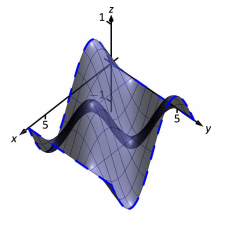8. $$f(x,y)=\frac{1}{x^2+y^2+1};\quad R$$ is the circle $$x^2+y^2=9$$.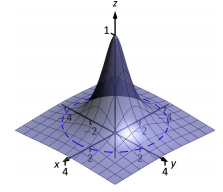9. $$f(x,y)=x^2-y^2;\quad R$$ is the rectangle with opposite corners $$(-1,-1)$$ and $$1,1)$$.10. $$f(x,y)=\frac{1}{e^{x^2}+1};\quad R$$ is the rectangle bounded by $$-5\le x \le 5$$ and $$0\le y \le 1$$.In Exercises 11-19, find the area of the given surface over the region R.

11. $$f(x,y)=3x-7y+2;\quad R$$ is the rectangle with opposite corners $$(-1,0)\text{ and }(1,3)$$.

12. $$f(x,y)=2x+2y+2;\quad R$$ is the triangle with corners $$(0,0),(1,0)\text{ and }(0,1)$$.

13. $$f(x,y)=x^2+y^2+10;\quad R$$ is the circle $$x^2+y^2=16$$.

14. $$f(x,y)=-2x+4y^2+7\text{ over } R$$, the triangle bounded by $$y=-x,y=x,0\le y \le 1$$.

15. $$f(x,y)=x^2+y$$ over R, the triangle bounded by $$y=2x,y=0 \text{ and }x=2$$.

16. $$f(x,y)=\frac{2}{3}x^{3/2}$$ over R, the rectangle with opposite corners $$(0,0)\text{ and }(1,1)$$.

17. $$f(x,y)=10-2\sqrt{x^2+y^2}$$ over R, the circle $$x^2+y^2=25$$. (This is the cone with height 10 and base radius 5; be sure to compare your result with the known formula.)

18. Find the surface area of the sphere with radius 5 by doubling the surface area of $$f(x,y)=\sqrt{25-x^2-y^2}$$ over R, the circle $$x^2+y^2=25$$. (Be sure to compare your result with the known formula.)

19. Find the surface area of the ellipse formed by restricting the plane $$f(x,y)=cx+dy+h$$ to the region R, the circle $$x^2+y^2=1$$, where c, d and h are some constants. Your answer should be given in terms of c and d; why does the value of h not matter?

## 13.6: Volume Between Surfaces and Triple Integration

### Terms and Concepts

1. The strategy for establishing bounds for triple integrals is "________ to ________, _________ and __________ to _______."

2. Give an informal interpretation of what $$"\int\int\int_D \,dV$$" means.

3. Give two uses of triple integration.

4. If an object has a constant density $$\delta$$ and a volume V, what is its mass?

### Problems

In Exercises 5-8, two surfaces $$f_1(x,y)$$ and $$f_2(x,y)$$ and a region R in the x,y plane are given. Set up and evaluate the double integral that finds the volume between these surfaces over R.

5. $$f_x(x,y) = 8-x^2-y^2,\,f_2(x,y) =2x+y;$$
R is the square with corners $$(-1,-1)\text{ and }(1,1)$$.

6. $$f_x(x,y) = x^2+y^2,\,f_2(x,y) =-x^2-y^2;$$
R is the square with corners $$(0,0)\text{ and }(2,3)$$.

7. $$f_x(x,y) = \sin x \cos y,\,f_2(x,y) =\cos x \sin y +2;$$
R is the triangle with corners $$(0,0), (\pi , 0)\text{ and }(\pi,\pi)$$.

8. $$f_x(x,y) = 2x^2+2y^2+3,\,f_2(x,y) =6-x^2-y^2;$$
R is the circle $$x^2+y^2=1$$.

In Exercises 9-16, a domain D is described by its bounding surfaces, along with a graph. Set up the triple integrals that give the volume of D in all 6 orders of integration, and find the volume of D by evaluating the indicated triple integral.

9. D is bounded by the coordinate planes and $$z=2-2x/3-2y$$.
Evaluate the triple integral with order dz dy dz.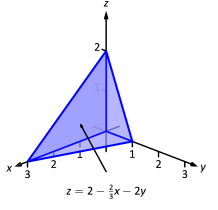10. D is bounded by the planes $$y=0,y=2,x=1,z=0\text{ and }z=(2-x)/2$$.
Evaluate the triple integral with order dx dy dz​​​​​​​.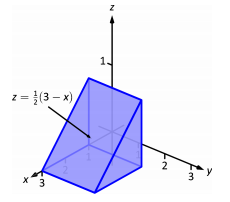11. D is bounded by the planes $$x=0,x=2,z=-y\text{ and by }z=y^2/2$$.
Evaluate the triple integral with order dy dz dx​​​​​​​.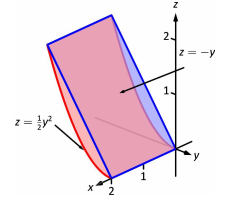12. D is bounded by the planes $$z=0,y=9, x=0\text{ and by$$z=\sqrt{y^2-9x^2}\).
Do not evaluate any triple integral.13. D is bounded by the planes $$x=2,y=1,z=0\text{ and }z=2x+4y-4$$.
Evaluate the triple integral with order dx dy dz​​​​​​​.14. D is bounded by the plane $$z=2y\text{ and by }y=4-x^2$$.
Evaluate the triple integral with order dz dy dz​​​​​​​.15. D is bounded by the coordinate planes and $$y=1-x^2\text{ and }y=1-z^2$$.
Do not evaluate any triple integral. Which order is easier to evaluate: dz dy dx or dy dz dx? Explain why.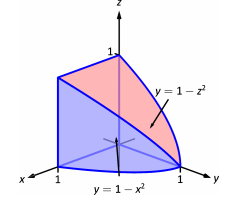16. D is bounded by the coordinate planes and by $$z=1-y/3\text{ and }z=1-x$$.
Evaluate the triple integral with order dx dy dz​​​​​​​.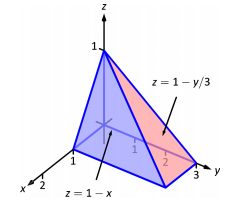In Exercises 17-20, evaluate the triple integral.

17. $$\int_{-\pi/2}^{\pi/2}\int_{0}^{\pi}\int_{0}^{\pi} (\cos x \sin y \sin z )dz\,dy\,dx$$

18. $$\int_{0}^{1}\int_{0}^{x}\int_{0}^{x+y} (x+y+z )dz\,dy\,dx$$

19. $$\int_{0}^{\pi}\int_{0}^{1}\int_{0}^{z} (\sin (yz))dx\,dy\,dz$$

20. $$\int_{\pi}^{\pi^2}\int_{x}^{x^3}\int_{-y^2}^{y^2} (\cos x \sin y \sin z )dz\,dy\,dx$$

In Exercises 21-24, find the center of mass of the solid represented by the indicated space region D with density function $$\delta (x,y,z)$$.

21. D is bounded by the coordinate planes and $$z=2-2x/3-2y$$; $$\delta (x,y,z)=10$$g/cm$$^3$$.
(Note: this is the same region as used in Exercise 9.)

22. D is bounded by the planes $$y=0,y=2,x=1,z=0 \text{ and }z=(3-x)/2$$; $$\delta (x,y,z)=2$$g/cm$$^3$$.
(Note: this is the same region as used in Exercise 10.)

23. D is bounded by the planes $$x=2,y=1,z=0\text{ and }z=2x+4y-4$$; $$\delta (x,y,z)=x^2$$lb/in$$^3$$.
(Note: this is the same region as used in Exercise 13.)

24. D is bounded by the planes $$z=2y\text{ and by }y=4-x^2$$. $$\delta (x,y,z)=y^2$$lb/in$$^3$$.
(Note: this is the same region as used in Exercise 14.)

14.E: Multiple Integration (Exercises) is shared under a CC BY-NC-SA license and was authored, remixed, and/or curated by LibreTexts.